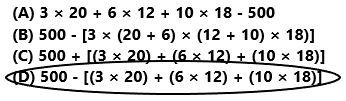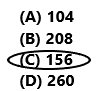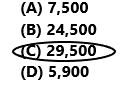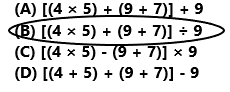# Texas Go Math Grade 5 Lesson 7.5 Answer Key Grouping Symbols

Refer to our Texas Go Math Grade 5 Answer Key Pdf to score good marks in the exams. Test yourself by practicing the problems from Texas Go Math Grade 5 Lesson 7.5 Answer Key Grouping Symbols.

## Texas Go Math Grade 5 Lesson 7.5 Answer Key Grouping Symbols

Unlock the Problem

Mary’s weekly allowance is $8 and David’s weekly allowance is$5. Every week they each spend $2 on lunch. Write a numerical expression to show how many weeks it will take them together to save enough money to buy a video game for$45.

• Underline Mary’s weekly allowance and how much she spends.
• Circle David’s weekly allowance and how much he spends.

Use parentheses and brackets to write an expression.

You can use parentheses and brackets to group operations that go together. Operations in parentheses and brackets are performed first.
STEP 1: Write an expression to represent how much Mary and David save each week.

• How much money does Mary save each week?
Think: Each week Mary gets $8 and spends$2.
(__________)
• How much money does David save each week?
Think: Each week David gets $5 and spends$2.
(__________)
• How much money do Mary and David save together each week? ___________

STEP 2: Write an expression to represent how many weeks it will take Mary and David to save enough money for the video game.

• How many weeks will it take Mary and David to save enough for a video game?
Think: I can use brackets to group operations a second time. $45 is divided by the total amount of money saved each week. _____________ ÷ [_______________] Answer: STEP 1: Each week Mary gets$8 and spends $2.$8 – $2$6

Each week Mary saves $6. Each week David gets$5 and spends $2.$5 – $2$3
Each week David saves $3. The expression to represent the how much Mary and David save each week is$6 + $3. Mary and David saves together each week is$9.
STEP 2:
In this step we are calculating how many weeks will it take Mary and David to save enough money for the video game.
Here we are using brackets to group operations a second time. $45 is divided by the total amount of money saved each week. The expression is$45 ÷ [$6 +$3]
$45 ÷$9
5
They took 5 weeks to save enough money to buy a video game.

Math Talk
Mathematical Processes

Explain why brackets are placed around the part of the expression that represents the amount of money Mary and David save each week.

Example

John gets $6 for his weekly allowance and spends$4 of it. His sister Tina gets $7 for her weekly allowance and spends$3 of it. Their mother’s birthday is in 4 weeks. If they save the same amount each week, how much money can they save together in that time to buy her a present?

• Write the expression using parentheses and brackets. 4 × [($6 –$4) + ($7 –$3)]
• Perform the operations in the parentheses first. 4 × [_______ + _______]
• Next perform the operations in the brackets. 4 × _______
• Then multiply. _______

So, John and Tina will be able to save _______ for their mother’s birthday present.
John gets $6 for his weekly allowance and spends$4 of it.
$6 –$4
His sister Tina gets $7 for her weekly allowance and spends$3 of it.
$7 –$3
Their mother’s birthday is in 4 weeks.
The expression using parentheses and brackets.
4 × [($6 –$4) + ($7 –$3)]
Perform the operations in the parentheses first.
4 × [$2 +$4]
Next perform the operations in the brackets.
4 × $6 Then multiply.$24
So, John and Tina will be able to save $24 for their mother’s birthday present. H.O.T. What if only Tina saves money? Will this change the numerical expression? Explain. Answer: If Tina only saves money then the numerical expression changes to 4 × ($7 – $3). Explanation: Tina gets$7 for her weekly allowance and spends $3 of it.$7 – $3 Their mother’s birthday is in 4 weeks. The expression using parentheses. 4 × ($7 – $3) Perform the operations in the parentheses first. 4 × ($4)
Then multiply.
$16 So, Tina will be able to save$16 for her mother’s birthday present.

Share and Show

Simplify the numerical expression.

Question 1.
12 + [(15 – 5) + (9 – 3)]
12 + [10 + ________]
12 + _______
12 + [(15 – 5) + (9 – 3)]
12 + [10 + 6]
12 + 16
28
Explanation:
The numerical expression using parentheses and brackets is 12 + [(15 – 5) + (9 – 3)]. Perform the operations in the parentheses first 12 + [10 + 6]. Next, perform the operations in the brackets 12 + 16. Then perform the addition operation the sum is 28. The simplified form of the given numerical expression is 28.

5 × [(26 – 4) – (4 + 6)]
5 × [(26 – 4) – (4 + 6)]
5 x [22 – 10]
5 x 12
60
Explanation:
The numerical expression using parentheses and brackets is 5 × [(26 – 4) – (4 + 6)]. Perform the operations in the parentheses first 5 x [22 -10]. Next perform the operations in brackets 5 x 12. Then perform multiplication operation the product is 60. The simplified form of the given numerical expression is 60.

Question 3.
36 ÷ [(18 – 10) – (8 – 6)]
36 ÷ [(18 – 10) – (8 – 6)]
36 ÷ [8 – 2]
36 ÷ 6
6
Explanation:
The numerical expression using parentheses and brackets is 36 ÷ [(18 – 10) – (8 – 6)]. Perform the operations in the parentheses first 36 ÷ [8 – 2]. Next, perform the operations in the brackets 36 ÷ 6. Then perform the division operation the result is 6. The simplified form of the given numerical expression is 6.

Problem Solving

Question 4.
H.O.T. Use Symbols Write the expression 2 × 8 + 20 – 12 + 6 with parentheses and brackets two different ways so its value is less than 10 and greater than 50.

Unlock the Problem

Question 5.
Reasoning Dan has a flower shop. Each day he displays 24 roses. He gives away 10 and sells the rest. Each day he displays 36 carnations. He gives away 12 and sells the rest. What expression can you use to find out how many roses and carnations Dan sells in a week?
a. What information are you given?
Dan has a flower shop. Each day he displays 24 roses. He gives away 10 and sells the rest. Each day he displays 36 carnations. He gives away 12 and sells the rest.

b. What are you being asked to do?
I was asked to find out how many roses and carnations Dan sells in a week.

c. What expression shows how many roses Dan sells in one day?
The expression is 24 – 10.
24 – 10
14
Dan sells 14 roses in one day.
d. What expression shows how many carnations Dan sells in one day?
The expression is 36 – 12.
36 – 12
24
Dan sells 24 carnations in one day.

e. Write an expression to represent the total number of roses and carnations Dan sells in one day.
(24 – 10) + (36 – 12)
14 + 24
38
Explanation:
The expression (24 – 10) + (36 – 12) represents the total number of roses and carnations Dan sells in one day is 38.

f. Write the expression that shows how many roses and carnations Dan sells in a week.
7 x [(24 – 10) + (36 – 12)]
Explanation:
The expression 7 x [(24 – 10) + (36 – 12)] represents the roses and carnations Dan sells in a week.

Multi-Step Simplify the expression to find out how many roses and carnations Dan sells in a week.
7 x [(24 – 10) + (36 – 12)]
7 x [14 + 24]
7 x 38
266
Dan sells 266 roses and carnations in a week.

Question 7.
H.O.T. How could you change the story in Problem 5 so there is only one expression inside parentheses?Question 8.
A gift shop had 500 colored pencils. The shop sold 3 sets of 20 colored pencils, 6 sets of 12 colored pencils, and 10 sets of 18 colored pencils. Which expression shows how many colored pencils are left?
(A) 3 × 20 + 6 × 12 + 10 × 18 – 500
(B) 500 – [3 × (20 + 6) × (12 + 10) × 18)]
(C) 500 + [(3 × 20) + (6 × 12) + (10 × 18)]
(D) 500 – [(3 × 20) + (6 × 12) + (10 × 18)]The expression is 500 – [(3 × 20) + (6 × 12) + (10 × 18)].
So, option D is correct.
Explanation:
A gift shop had 500 colored pencils. The shop sold 3 sets of 20 colored pencils, 6 sets of 12 colored pencils, and 10 sets of 18 colored pencils. The expression that shows the colored pencils left is 500 – [(3 × 20) + (6 × 12) + (10 × 18)]. So, option D is correct.

Question 9.
Anya buys 8 oranges every Monday morning at the farmer’s market. She gives 6 away and eats the rest. Every Friday she buys 6 oranges, gives 5 away, and eats the rest. Simplify the expression 52 × [(8 – 6) + (6 – 5)] to find the number of oranges Anya eats in a year.
(A) 104
(B) 208
(C) 156
(D) 26052 × [(8 – 6) + (6 – 5)]
52 x [2 + 1]
52 x 3
156
Anya eats 156 oranges in a year.
So, option C is correct.
Explanation:
Anya buys 8 oranges every Monday morning at the farmer’s market. She gives 6 away and eats the rest. Every Friday she buys 6 oranges, gives 5 away, and eats the rest. The expression is 52 × [(8 – 6) + (6 – 5)]. The simplified form of the expression value is 156. Anya eats 156 oranges in a year. So, option C is correct.

Question 10.
Multi-Step A company can produce 300 ballpoint pens or 550 gel pens each hour. Each weekday, the company produces ballpoint pens for 5 hours and gel pens for 8 hours. How many pens does the company produce in 5 weekdays?
(A) 7,500
(B) 24,500
(C) 29,500
(D) 5,900The expression is 5 x [(5 x 300) + (8 x 550)].
5 x [1,500 + 4,400]
5 x 5,900
29,500
The company produce 29,500 pens in 5 weekdays.
So, option C is correct.
Explanation:
A company can produce 300 ballpoint pens or 550 gel pens each hour. Each weekday, the company produces ballpoint pens for 5 hours and gel pens for 8 hours. The expression is 5 x [(5 x 300) + (8 x 550)]. The simplified form of the expression value is 29,500. The company produces 29,500 pens in 5 weekdays.
So, option C is correct.
Texas Test Prep

Which expression has a value of 4?
(A) [(4 × 5) + (9 + 7)] + 9
(B) [(4 × 5) + (9 + 7)] ÷ 9
(C) [(4 × 5) – (9 + 7)] × 9
(D) [(4 + 5) + (9 + 7)] – 9[(4 × 5) + (9 + 7)] ÷ 9
[20 + 16] ÷ 9
36 ÷ 9
4
Explanation:
The numerical expression using parentheses and brackets is [(4 × 5) + (9 + 7)] ÷ 9. Perform the operations in the parentheses first [20 + 16] ÷ 9. Next perform the operations in the brackets 36 ÷ 9. Then perform division operation the result is 4. The expression that has a value of 4 is [(4 × 5) + (9 + 7)] ÷ 9.

### Texas Go Math Grade 5 Lesson 7.5 Homework and Practice Answer Key

Simplify the numerical expression.

Question 1.
14 + [(2 × 5) + (3 × 8)]
14 + [(2 × 5) + (3 × 8)]
14 + [10 + 24]
14 + 34
48
Explanation:
The numerical expression using parentheses and brackets is 14 + [(2 × 5) + (3 × 8)]. Perform the operations in the parentheses first 14 + [10 + 24]. Next perform the operations in the brackets 14 + 34. Then perform addition operation the result is 48. The simplified form of given numerical expression is 48.

Question 2.
5 × [(8 + 2) – (16 – 9)]
5 × [(8 + 2) – (16 – 9)]
5 x [10 – 7]
5 x 3
15
Explanation:
The numerical expression using parentheses and brackets is 5 × [(8 + 2) – (16 – 9)]. Perform the operations in the parentheses first 5 × [10 – 7]. Next perform the operations in the brackets 5 x 3. Then perform multiplication operation the result is 15. The simplified form of given numerical expression is 15.

Question 3.
40 ÷ [(18 – 9) – (13 – 12)]
40 ÷ [(18 – 9) – (13 – 12)]
40 ÷ [9 – 1]
40 ÷ 8
5
Explanation:
The numerical expression using parentheses and brackets is 40 ÷ [(18 – 9) – (13 – 12)]. Perform the operations in the parentheses first 40 ÷ [9 – 1]. Next, perform the operations in the brackets 40 ÷ 8. Then perform the division operation the result is 5. The simplified form of the given numerical expression is 5.

Go Math Practice and Homework Lesson 7.5 Answer Key Question 4.
[(15 + 5) + (5 × 2)] ÷ 3
[(15 + 5) + (5 × 2)] ÷ 3
[20 + 10] ÷ 3
30 ÷ 3
10
Explanation:
The numerical expression using parentheses and brackets is [(15 + 5) + (5 × 2)] ÷ 3. Perform the operations in the parentheses first [20 + 10] ÷ 3. Next perform the operations in the brackets 30 ÷ 3. Then perform division operation the result is 10. The simplified form of given numerical expression is 10.

Question 5.
[(21 – 13) + (32 – 24)] × 4
[(21 – 13) + (32 – 24)] × 4
[8 + 8] × 4
16 x 4
64
Explanation:
The numerical expression using parentheses and brackets is [(21 – 13) + (32 – 24)] × 4. Perform the operations in the parentheses first [8 + 8] x 4. Next perform the operations in the brackets 16 x 4. Then perform multiplication operation the result is 64. The simplified form of given numerical expression is 64.

Question 6.
49 – [(3 × 4) + (9 × 2)]
49 – [(3 × 4) + (9 × 2)]
49 – [12 + 18]
49 – 30
19
Explanation:
The numerical expression using parentheses and brackets is 49 – [(3 × 4) + (9 × 2)]. Perform the operations in the parentheses first 49 – [12 + 18]. Next perform the operations in the brackets 49 – 30. Then perform subtraction operation the result is 19. The simplified form of given numerical expression is 19.

Question 7.
32 + [(11 – 7) + (5 × 3)]
32 + [(11 – 7) + (5 × 3)]
32 + [4 + 15]
32 + 19
51
Explanation:
The numerical expression using parentheses and brackets is 32 + [(11 – 7) + (5 × 3)]. Perform the operations in the parentheses first 32 + [4 + 15]. Next perform the operations in the brackets 32+ 19. Then perform addition operation the result is 51. The simplified form of given numerical expression is 51.

Question 8.
[(6 × 9) – (7 × 4)] – 17
[(6 × 9) – (7 × 4)] – 17
[54 – 28] – 17
26 – 17
9
Explanation:
The numerical expression using parentheses and brackets is [(6 × 9) – (7 × 4)] – 17. Perform the operations in the parentheses first [54 – 28] – 17. Next perform the operations in the brackets 26 – 17. Then perform subtraction operation the result is 9. The simplified form of given numerical expression is 9.

Question 9.
[(13 – 9) × 3] + [(14 – 8) × 2]
[(13 – 9) × 3] + [(14 – 8) × 2]
[4 × 3] + [6 × 2]
12 + 12
24
Explanation:
The numerical expression using parentheses and brackets is [(13 – 9) × 3] + [(14 – 8) × 2]. Perform the operations in the parentheses first [4 × 3] + [6 × 2]. Next perform the operations in the brackets 12 + 12. Then perform addition operation the result is 24. The simplified form of given numerical expression is 24.

Question 10.
[(2 × 6) + 3] + [35 – (7 × 3)]
[(2 × 6) + 3] + [35 – (7 × 3)]
[12 + 3] + [35 – 21]
15 + 14
29
Explanation:
The numerical expression using parentheses and brackets is [(2 × 6) + 3] + [35 – (7 × 3)]. Perform the operations in the parentheses first [12 + 3] + [35 – 21]. Next perform the operations in the brackets 15 + 14. Then perform addition operation the result is 29. The simplified form of given numerical expression is 29.

Problem Solving

Question 11.
Fred’s Car Dealership has a three-floor parking garage with cars for sale. Each floor has 3 rows of 5 compact cars and 4 rows of 8 sedans. Write an expression you can use to find the number of cars in Fred’s garage. Simplify the expression.
3 x [(3 x 5) + (4 x 8)]
3 x [15 + 32]
3 x 47
141
The number of cars in Fred’s garage is 141.
Explanation:
Fred’s Car Dealership has a three-floor parking garage with cars for sale. Each floor has 3 rows of 5 compact cars and 4 rows of 8 sedans. The expression is 3 x [(3 x 5) + (4 x 8)]. The number of cars in Fred’s garage is 141.

Question 12.
A carpenter has a supply of 84 large and small boards for the cabinets he builds. One week, he uses 3 sets of 8 small boards. Then he buys 3 more sets of 6 large boards. Write an expression you can use to find the number of boards the carpenter has now. Simplify the expression.

Lesson Check

Question 13.
Which expression has a value of 1?
(A) 30 + [(9 × 2) + (4 × 3)]
(B) 30 ÷ [(9 × 2) + (4 × 3)]
(C) 30 – [(9 × 2) + (4 × 3)]
(D) 30 × [(9 + 2) – (4 + 3)]30 ÷ [(9 × 2) + (4 × 3)]
30 ÷ [18 + 12]
30 ÷ 30
1
So, option B is correct.
Explanation:
The expression that has the value of 1 is 30 ÷ [(9 × 2) + (4 × 3)]. Perform the operations in the parentheses first 30 ÷ [18 + 12]. Next, perform the operations in the brackets 30 ÷ 30. Then perform the division operation the value is 1.

Which expression has a value equal to the value of the expression 4 × [(12 + 4) – (12 ÷ 3)]?
(A) (4 × 12) + 4
(B) 4 × (16 – 4)
(C) 4 + (12 × 4)
(D) 4 × 12 + 44 × [(12 + 4) – (12 ÷ 3)]
4 x [16 – 4]
4 x 12
48
So, option B is correct.
Explanation:
The value of this expression 4 x (16 – 4) is 48. The value of this expression 4 × [(12 + 4) – (12 ÷ 3)] is 48. So, the expression 4 x (16 – 4) has a value  is equal to the value of the expression 4 × [(12 + 4) – (12 ÷ 3)]. So, option B is correct.

Question 15.
The school math coach takes an inventory of math materials. He counts the materials for 5 different classes. Each class has 7 boxes of 10 pattern blocks, 6 boxes of 9 rulers, and 3 boxes of 2 calculators. Which expression shows the number of math materials?
(A) 5 × [(7 × 10) + (6 × 9) + (3 × 2)]
(B) 5 + [(7 × 10) + (6 × 9) + (3 × 2)]
(C) 5 × 7 × 10 + 6 × 9 + 3 × 2
(D) [(7 × 10) + (6 × 9) + (3 × 2)] – 5option A is correct.
Explanation:
The school math coach takes an inventory of math materials. He counts the materials for 5 different classes. Each class has 7 boxes of 10 pattern blocks, 6 boxes of 9 rulers, and 3 boxes of 2 calculators. The expression that shows the number of math materials is 5 × [(7 × 10) + (6 × 9) + (3 × 2)]. So, option A is correct.

Question 16.
At the beginning of the year, the teacher’s supply closet contained 250 markers. In September, 5 sets of 6 black markers, 4 sets of 5 red markers, and 6 sets of 3 yellow markers are used. Which expression shows the number of markers left?
(A) 250 + [(5 × 6) + (4 × 5) + (6 × 3)]
(B) (250 – 5) × 6 + [(4 × 5) + (6 × 3)]
(C) 250 + [(5 + 6) × (4 + 5) × (6 + 3)]
(D) 250 – [(5 × 6) + (4 × 5) + (6 × 3)]Option D is correct.
Explanation:
At the beginning of the year, the teacher’s supply closet contained 250 markers. In September, 5 sets of 6 black markers, 4 sets of 5 red markers, and 6 sets of 3 yellow markers are used. The expression that shows the number of markers left is 250 – [(5 × 6) + (4 × 5) + (6 × 3)].

Question 17.
Multi-Step The principal conducted a school assembly every school day for a week. On Monday, 78 students attended. Then 6 classes with 25 students in each class attended each day for the next three days. On Friday, 8 classes with 32 students in each class attended the assembly. How many students attended the assembly?
(A) 606
(B) 484
(C) 706
(D) 78478 + 3 x (6 x 25) + (8 x 32)
78 + 3 x 150 + 256
78 + 450 + 256
784
So, option D is correct.
Explanation:
The principal conducted a school assembly every school day for a week. On Monday, 78 students attended. Then 6 classes with 25 students in each class attended each day for the next three days. On Friday, 8 classes with 32 students in each class attended the assembly. 784 students attended the assembly. So, option D is correct.

Question 18.
Multi-Step Employees from a local store donated picnic supplies for the end-of-the-school-year picnic. They donated 20 packs of 12 forks, 10 packs of 12 spoons, 5 packs of 10 knives, and 175 paper plates. How many picnic items were donated?
(A) 244
(B) 410
(C) 585
(D) 695